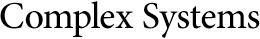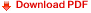## Mathematical Properties of Thermodynamic AutomataGuido Cosenza
Dipartimento di Scienze Fisiche, Universita di Napoli,
Mostra d'Oltremare, Padiglione 19, I-80125 Napoli, Italy

Anthony Massobrio

Luigi Sertorio
Present address: Service de Chimie Physique, ULB, Bruxelles, Belgium.

Dipartimento di Fisica Teorica dell'Universita di Torino, INFN,
Via P. Giuria 1, I-10125 Torino, Italy

#### Abstract

This paper concerns the study of some mathematical properties of Thermodynamic Automata. In the present paper, the governing linearized differential equations---for which a rigorous treatment is possible---are studied. The driving terms are assumed periodic and the controls are assumed piecewise constant. The explicit solution is found in the most general case with an arbitrary number of compartments composing the Thermodynamic Automaton and it is shown to be periodic with the same period of the driving terms. This property allows the derivation of interesting relationships among the time averages of the temperatures. Moreover, knowledge of the solutions enables proof that the trilinear system presented here and a bilinear system of equations introduced in a preceding paper are equivalent.